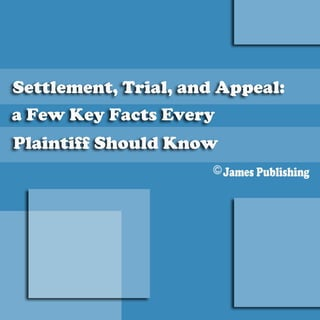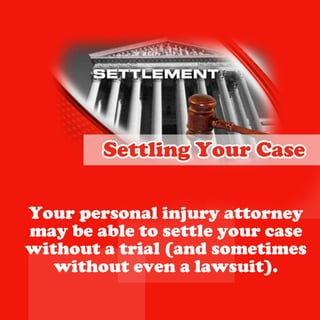Ce diaporama a bien été signalé.
Le téléchargement de votre SlideShare est en cours. ×

# Settlement, Trial, and Appeal a Few Key Facts Every Plaintiff Should Know

Publicité
Publicité
Publicité
Publicité
Publicité
Publicité
Publicité
Publicité
Publicité
Publicité
PublicitéPublicité
Publicité
Chargement dans…3
×

## Consultez-les par la suite

1 sur 12 Publicité

# Settlement, Trial, and Appeal a Few Key Facts Every Plaintiff Should Know

As you begin your personal injury case, here are some basic facts about settlement offers, trial, post-trial motions, and appeals that you should understand.

Your personal injury attorney may be able to settle your case without a trial (and sometimes without even a lawsuit).

You Have a Right to Reject Any Settlement Offer
Your attorney cannot settle your case unless you approve and consent. If you are not satisfied with the settlement offer, you have a right to let the jury decide your case.

As you begin your personal injury case, here are some basic facts about settlement offers, trial, post-trial motions, and appeals that you should understand.

Your personal injury attorney may be able to settle your case without a trial (and sometimes without even a lawsuit).

You Have a Right to Reject Any Settlement Offer
Your attorney cannot settle your case unless you approve and consent. If you are not satisfied with the settlement offer, you have a right to let the jury decide your case.

Publicité
Publicité

### Settlement, Trial, and Appeal a Few Key Facts Every Plaintiff Should Know

1. 1. S tlme tT ila dAp e l ete n , ra, n p a: aF w Ke F cs v r e y a t E ey P an i S o l Kn w litf h u d o f J me P bihn a s u l ig s
2. 2. S tl gY u C s eti n o r ae Y u p ro a i j r a t r e o r e s n ln u y to n y ma b a l t stl y u c s y e be o ete o r a e wih u atil a ds mei s t o t ra ( n o tme wih u e e alws i) t o t v n a u t.
3. 3. Y uHa e R g t o o v a ih t R jc An S tlme t ee t y ete n O fr fe Y u at r e c n o stl o r to n y a n t ete y u c s u ls y ua p o e o r a e n es o p r v a dc n e t I y ua e o n o sn . f o r n t s tsidwiht e ete n aif e t h stlme t ofr y uh v arg t olt fe , o a e ih t e t e u yd cd y u c s. h j r e ie o r a e
4. 4. S Do s h Dee d n o e t e fn a t B t e a tk n t e y h s me o e ,h d fn a t loh s rg t oa ee d n as a a ih t tila dy u at r e c n o ra, n o r to n y a n t fr e h d fn a t ostl o c t e ee d n t ete t e ae h c s.
5. 5. Do ’B T oE g r oS tl nt e o a e t ete B a i midt a i y ua p a e r n n h tf o p e r t oe g r ostl, o a e i ey o a e t ete y u r l l k t d c e s t e au o y u c s o e r ae h v le f o r ae i t e y s f h d fn a tT e n h e e o t e ee d n . h itl g n e au to a d n el e t v l ain n i stlme t f c s c l fr u t ete n o a a e al o j s s a h g ad g e o lg l k la a s ih e r e f e a s i s l til n ,f o e r p ry otn ra a d i d n p o e l, fe tk s u t s c tme a e j s a mu h i .
6. 6. I Y u C s G e t T il f o r a e o s o ra I y u c s c n o b stld i wi f o r a e a n t e ete ,t l l h v t b tid Mo t f h tme a e o e re . s o t e i , t e ra wi b b fr aj r .f o h til l e eo e u y I y u l tsi , o wi b a k dt tlt e etf y u l e s e o el h y l j d e n j r wh t o k o u g aduy a y u n w. O h r t ess ltsi a wel t e win se wi etf s l l y , a de ie c s c a r c r s n n vd n e u h s e o d a d d c me t wi b ito u e . h o u n s l e n r d cd T e l j r wi ma e d cso , n b sd uy l l k a e iin a d a e o t a d cso ,h j d e l n h t e iin t e u g wi l e tr j d me tet e fr r n e a u g n , ih r o o a an t o . g is y u
7. 7. T ee e h r Ar No G a a te u r n es Y u at r e n t r l h p s o r to n y au al o e y t wi y u c s, u c n o o n o r ae b t a n t g a a te n p riu a u r n e a y a tc lr r s l. u y u at r e eu t B t o r to n y wo l n t a e a e y u u d o h v tk n o r c s i h o s e i n t eiv a e f e r h dd o b l e e y uh v ar a o a l c a c o a e e s n be h n e t wi o o ti ag o o n r b an o d stlme t ete n .
8. 8. I Y uWi Afe aT il f o n tr ra I y uwi y u c s,h fo n o r ae t e d fn a t a et e p yt e ee d n c n ih r a h v r it r i amo infr e dc o f e l to o a n w til e ra.
9. 9. I Y uL s Afe aT il f o o e tr ra I y uls, o h v t e a f o o e y u a e h s me c oc :oa c p t e e dc o h ie t c e t h v r it r t c aln e t yma iga o h l g ib e kn mo infr n w til to o a e ra.
10. 10. It e u g O d r f h J d e r es aNe T il w ra T e ra j d e yd cd t gv h tilu g ma e ie o ie t e o igsd ( eh r o o t e h lsn ie wh t e y u r h d fn a t an w tilf h f s ee d n ) e ra i t e i t r til s o fi o i t e ra j d e ra wa n t ar r f h tilu g ma e e a mitk s h t k i d lg l sa e t a ma e t rg t og a t h lsr n w til ih t r n t e o e a e ra. E e i an w tils r e e ,h r v n f e ra i o d r d t e e i n a s r n e h t sc n til s o su a c t a a e o d ra wi h v adfe e t r etr l a e i r n o b te l f o to . u c me
11. 11. I t e u g R f ss o f h J d e eu e t O d r Ne T il r e a w ra I t e u g d ne t e to f h j d e e is h mo in fr n w tilt e a t o a e ra,h p ry se igt e e til wh t e e k n h n w ra ( eh r y uo t e ee d n ) a o r h d fn a t c n a p a. p el
12. 12. I Y u C s I Ap e ld f o r a e s p ae Ma yp o l t ikt a a n e pe hn h t n a p a a t maial e tte t e p e l u o tc l n ils h y lsr oasc n tilT i i n t oe t e o d ra. h s s o tu .n ta o g a tn an w r e I se d f r n ig e tila a p as o r ma o d r ra, n p e l c u t y r e o l as lc a g o n c a g n y mal h n e r o h n e i t e u g n g a tdb t e n h j d me t r n e y h tilu g . ra j d e